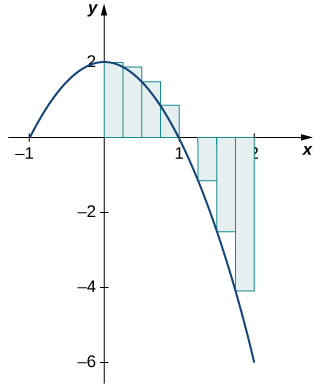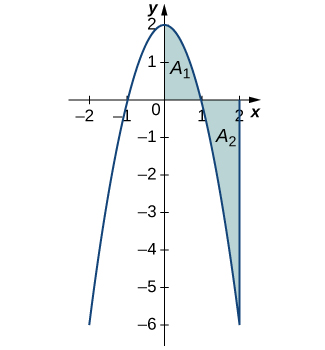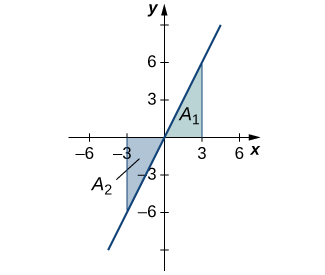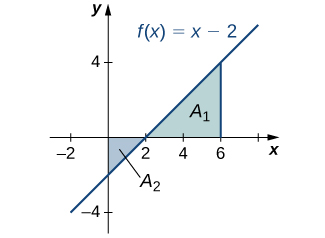# 5.2 The definite integral  (Page 3/16)

 Page 3 / 16

Use the formula for the area of a trapezoid to evaluate ${\int }_{2}^{4}\left(2x+3\right)dx.$

18 square units

## Area and the definite integral

When we defined the definite integral, we lifted the requirement that $f\left(x\right)$ be nonnegative. But how do we interpret “the area under the curve” when $f\left(x\right)$ is negative?

## Net signed area

Let us return to the Riemann sum. Consider, for example, the function $f\left(x\right)=2-2{x}^{2}$ (shown in [link] ) on the interval $\left[0,2\right].$ Use $n=8$ and choose $\left\{{x}_{i}^{*}\text{}}$ as the left endpoint of each interval. Construct a rectangle on each subinterval of height $f\left({x}_{i}^{*}\right)$ and width Δ x . When $f\left({x}_{i}^{*}\right)$ is positive, the product $f\left({x}_{i}^{*}\right)\text{Δ}x$ represents the area of the rectangle, as before. When $f\left({x}_{i}^{*}\right)$ is negative, however, the product $f\left({x}_{i}^{*}\right)\text{Δ}x$ represents the negative of the area of the rectangle. The Riemann sum then becomes

$\sum _{i=1}^{8}f\left({x}_{i}^{*}\right)\text{Δ}x=\left(\text{Area of rectangles above the}\phantom{\rule{0.2em}{0ex}}x\text{-axis}\right)-\left(\text{Area of rectangles below the}\phantom{\rule{0.2em}{0ex}}x\text{-axis}\right)$For a function that is partly negative, the Riemann sum is the area of the rectangles above the x -axis less the area of the rectangles below the x -axis.

Taking the limit as $n\to \infty ,$ the Riemann sum approaches the area between the curve above the x -axis and the x -axis, less the area between the curve below the x -axis and the x -axis, as shown in [link] . Then,

$\begin{array}{cc}{\int }_{0}^{2}f\left(x\right)dx\hfill & =\underset{n\to \infty }{\text{lim}}\sum _{i=1}^{n}f\left({c}_{i}\right)\text{Δ}x\hfill \\ & ={A}_{1}-{A}_{2}.\hfill \end{array}$

The quantity ${A}_{1}-{A}_{2}$ is called the net signed area    .In the limit, the definite integral equals area A 1 less area A 2 , or the net signed area.

Notice that net signed area can be positive, negative, or zero. If the area above the x -axis is larger, the net signed area is positive. If the area below the x -axis is larger, the net signed area is negative. If the areas above and below the x -axis are equal, the net signed area is zero.

## Finding the net signed area

Find the net signed area between the curve of the function $f\left(x\right)=2x$ and the x -axis over the interval $\left[-3,3\right].$

The function produces a straight line that forms two triangles: one from $x=-3$ to $x=0$ and the other from $x=0$ to $x=3$ ( [link] ). Using the geometric formula for the area of a triangle, $A=\frac{1}{2}bh,$ the area of triangle A 1 , above the axis, is

${A}_{1}=\frac{1}{2}3\left(6\right)=9,$

where 3 is the base and $2\left(3\right)=6$ is the height. The area of triangle A 2 , below the axis, is

${A}_{2}=\frac{1}{2}\left(3\right)\left(6\right)=9,$

where 3 is the base and 6 is the height. Thus, the net area is

${\int }_{-3}^{3}2xdx={A}_{1}-{A}_{2}=9-9=0.$The area above the curve and below the x -axis equals the area below the curve and above the x -axis.

Analysis

If A 1 is the area above the x -axis and A 2 is the area below the x -axis, then the net area is ${A}_{1}-{A}_{2}.$ Since the areas of the two triangles are equal, the net area is zero.

Find the net signed area of $f\left(x\right)=x-2$ over the interval $\left[0,6\right],$ illustrated in the following image.6

## Total area

One application of the definite integral is finding displacement when given a velocity function. If $v\left(t\right)$ represents the velocity of an object as a function of time, then the area under the curve tells us how far the object is from its original position. This is a very important application of the definite integral, and we examine it in more detail later in the chapter. For now, we’re just going to look at some basics to get a feel for how this works by studying constant velocities.

Find the derivative of g(x)=−3.
any genius online ? I need help!!
Pina
need to learn polynomial
Zakariya
i will teach...
nandu
I'm waiting
Zakariya
plz help me in question
Abish
Tlou
evaluate the following computation (x³-8/x-2)
teach me how to solve the first law of calculus.
what is differentiation
f(x) = x-2 g(x) = 3x + 5 fog(x)? f(x)/g(x)
fog(x)= f(g(x)) = x-2 = 3x+5-2 = 3x+3 f(x)/g(x)= x-2/3x+5
diron
pweding paturo nsa calculus?
jimmy
how to use fundamental theorem to solve exponential
find the bounded area of the parabola y^2=4x and y=16x
what is absolute value means?
Chicken nuggets
Hugh
🐔
MM
🐔🦃 nuggets
MM
(mathematics) For a complex number a+bi, the principal square root of the sum of the squares of its real and imaginary parts, √a2+b2 . Denoted by | |. The absolute value |x| of a real number x is √x2 , which is equal to x if x is non-negative, and −x if x is negative.
Ismael
find integration of loge x
find the volume of a solid about the y-axis, x=0, x=1, y=0, y=7+x^3
how does this work
Can calculus give the answers as same as other methods give in basic classes while solving the numericals?
log tan (x/4+x/2)
Rohan
Rohan
y=(x^2 + 3x).(eipix)
Claudia
Ismael
A Function F(X)=Sinx+cosx is odd or even?
neither
David
Neither
Lovuyiso
f(x)=1/1+x^2 |=[-3,1]
apa itu?
fauzi

#### Get Jobilize Job Search Mobile App in your pocket Now!By Yacoub JayoghliBy Alec MoffitBy OpenStaxBy Brooke DelaneyBy OpenStaxBy Carly AllenBy Anonymous UserBy OpenStaxByBy OpenStax